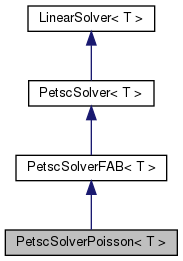# PetscSolverPoisson< T > Class Template Reference

`#include <PetscSolver.H>`

Inheritance diagram for PetscSolverPoisson< T >:[legend]

## Public Member Functions

PetscSolverPoisson ()
virtual void define (Real a_dx, bool a_homogeneous=true)
virtual void define (LinearOp< T > *a_op, bool a_homogeneous=true)
virtual int formMatrix (Mat, const LevelData< FArrayBox > *=0, PetscInt my0=0, PetscInt nloc=0, PetscInt *d=0, PetscInt *o=0)

Real m_alpha
Real m_beta

## Constructor & Destructor Documentation

template<class T>
 PetscSolverPoisson< T >::PetscSolverPoisson ( )

## Member Function Documentation

template<class T>
 virtual void PetscSolverPoisson< T >::define ( Real a_dx, bool a_homogeneous = `true` ) ` [virtual]`

Reimplemented from PetscSolver< T >.

template<class T>
 virtual void PetscSolverPoisson< T >::define ( LinearOp< T > * a_operator, bool a_homogeneous = `true` ) ` [inline, virtual]`

Define the operator and whether it is a homogeneous solver or not. The LinearSolver does not take over ownership of this a_operator object. It does not call delete on it when the LinearSolver is deleted. It is meant to be like a late-binding reference. If you created a_operator with new, you should call delete on it after LinearSolver is deleted if you want to avoid memory leaks.

Reimplemented from PetscSolver< T >.

References PetscSolver< T >::define().

template<class T>
 virtual int PetscSolverPoisson< T >::formMatrix ( Mat , const LevelData< FArrayBox > * = `0`, PetscInt my0 = `0`, PetscInt nloc = `0`, PetscInt * d = `0`, PetscInt * o = `0` ) ` [virtual]`

## Member Data Documentation

template<class T>
 Real PetscSolverPoisson< T >::m_alpha

template<class T>
 Real PetscSolverPoisson< T >::m_beta

The documentation for this class was generated from the following file:

Generated on Mon Mar 25 03:28:01 2019 for Chombo + EB + MF by1.5.5